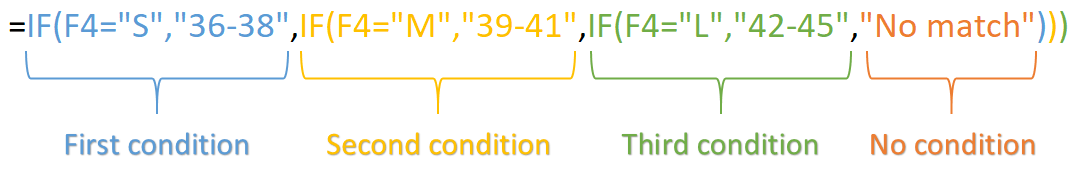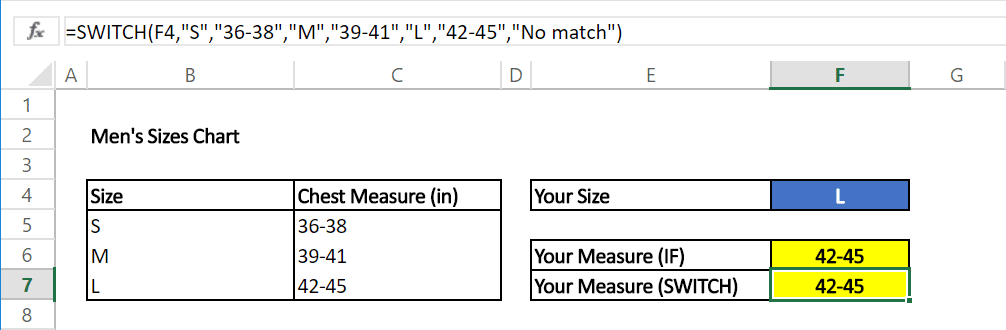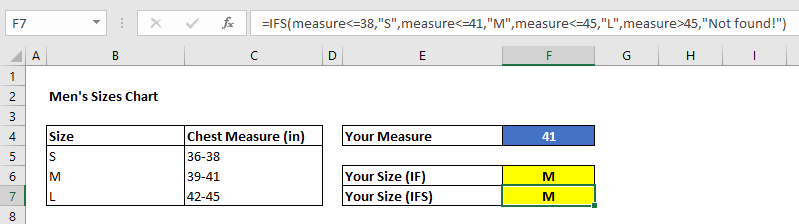Nested IF statements are useful for creating more complicated scenarios in Excel. However, IFS and SWITCH formulas can work more efficiently when dealing with more than one condition. These formulas work a bit differently than the legacy IF function. Let's start with the basics.

To perform a logical test, the IF function is usually the go-to method. However, due to its parameter structure, it poses a flaw when it comes to applying more than one logical tests. You need to use nested IF statements in such cases. This can prove challenging to create, read, and maintain. For example;The IFS and the SWITCH functions can handle this type of situations easier. Both functions has been released in Excel 2016 via Office 365. Each function addresses different pieces of issues stemming from nested IF statements.

The IFS function allows building multiple conditions. On the other hand, the SWITCH function allows only to entering one condition. If you want to return different results based on an expression, use the SWITCH function. If you have different expressions for each level, use the IFS function. Another advantage of the IFS function is that it allows using logic operators like greater than (>) or less than (<) in the expression. The SWITCH function only allows exact matching.

# SWITCH

The SWITCH function evaluates the value in its first argument against the value-result pairs, and returns the result from the matched pair if there is a match or default value if there isn't. Although you can use up to 126 value-result pairs, we do not recommend this use case, and instead using the VLOOKUP function for matching large condition set. For more information about the VLOOKUP function, see HOW TO VLOOKUP.

## Syntax

=SWITCH(expression, value1, result1, [default or value2, result2],…[default or value3, result3])

Here is a sample use case of the SWITCH function:The SWITCH function checks the size in cell F4 and returns the corresponding value.# IFS

The IFS function evaluates multiple conditions until it finds the first condition that gives a TRUE result. As the name suggests, it is designed to replace nested IF statements containing multiple IF functions. The IFS function's arguments are test-value pairs.

## Syntax

=IFS(logical_test1, value_if_true1, [logical_test2, value_if_true2], [logical_test3, value_if_true3],…)

Let's see how it works in the examples above.In our example, the IFS function checks 4 different logical tests in order and returns the corresponding value when it reaches a test result of TRUE. To do the same, you would need 4 different nested IF statements, or a SWITCH function with 20 value-result pairs (36 - 45).